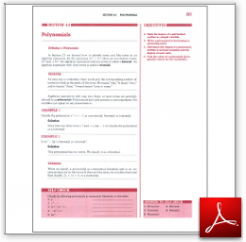Algebra Math Book - Introductory Algebra Thank you for your continual support.

 The power of mathematics rests in the logic of thinking.ID: Sec4-2
Description: Defining a Polynomial-Degree of a Polynomial in One Variable-Several Variables-Order-Evaluating
Price: 1.99

# Algebra Math book - Introductory Algebra - Chapter 4 - Section 2 - Polynomials

## Section 4.2 - Objectives

3.  State the degree of a polynomial written in a single variable.

4.  Write a polynomial in decreasing or ascending order.

5.  Determine the degree of a polynomial written in several variables and the degree of each term.

6.  Find the value of a polynomial given specific values for the variable(s).

Navigate to
Previous Section or Next Section
or Chapter 4 Details or Other Chapters

This section of my algebra math book, Introductory Algebra, also includes in the download:

• Cover Sheet